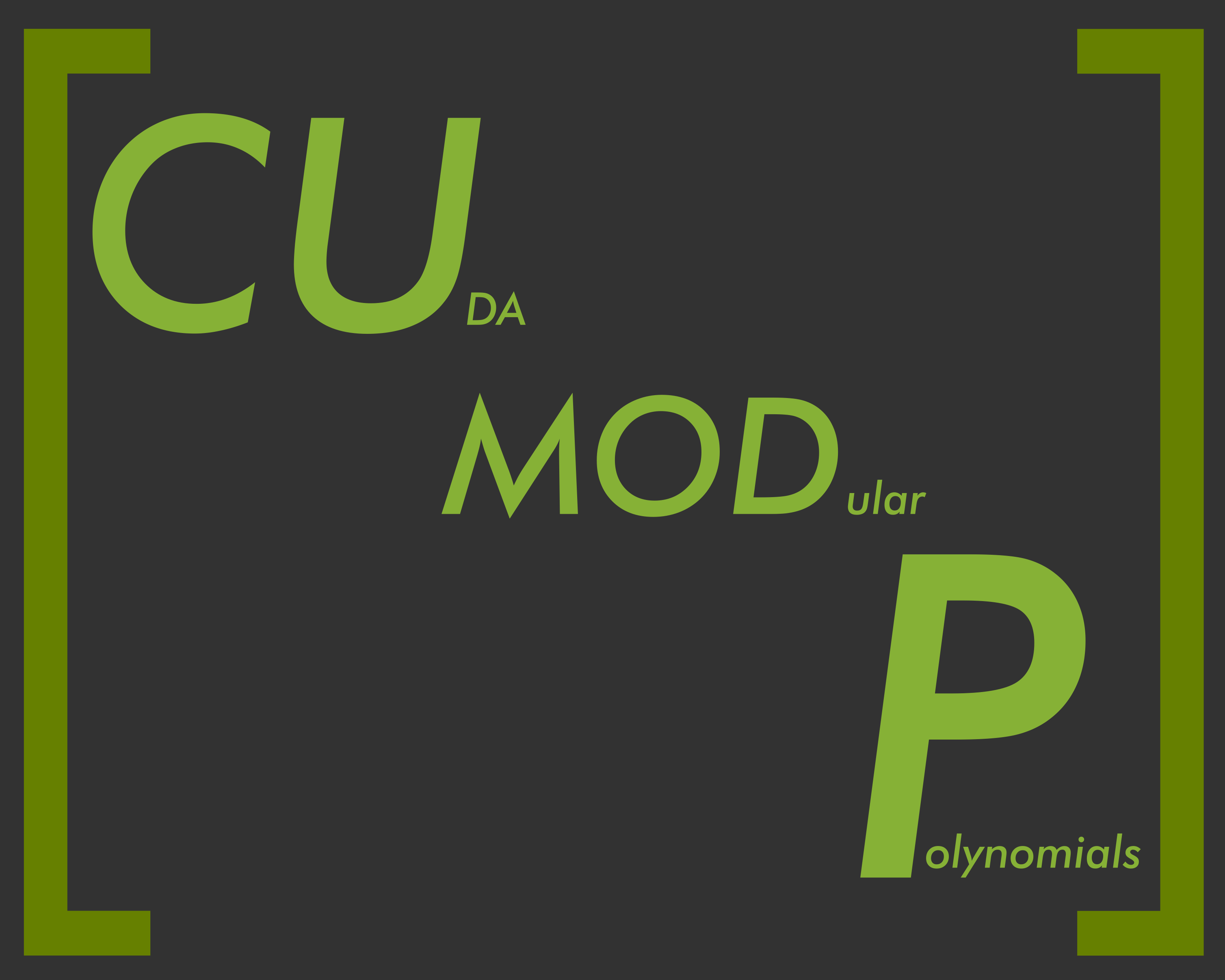A CUDA Library for Modular Polynomial Computation

The CUDA Modular Polynomial (CUMODP) Library implements arithmetic operations for dense matrices and dense polynomials, primarily with modular integer coefficients. Some operations are available for integer or floating point coefficients.

For the moment, following operations are implemented in CUMODP library:

• Matrix determinant computation
• Polynomial multiplication (both plain and FFT-based)
• Univariate polynomial division
• The Euclidean algorithm for computing GCD of univariate polynomials
• Subproduct tree techniques for multi-point evaluation and interpolation
• Fast Fourier transform (GPU implementation of Cooley-Tukey and Stockham FFT algorithms)
• Big prime field FFT
• Subresultant chain computation for multivariate polynomials
• Bivariate system solving

The main CUMODP Library target GPU machines and is written in CUDA C++ .

Also, the library includes a supporting C library called MODPN.

If you have questions or bug reports, please contact: cumodp sdl [dot] csd [dot] uwo [dot] ca